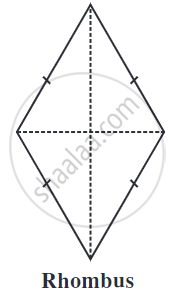# Types of Quadrilaterals - Properties of Rhombus

#### definition

Rhombus: A rhombus is a quadrilateral with four equal-length sides and opposite sides parallel to each other.

# Rhombus:

A rhombus is a quadrilateral with four equal-length sides and opposite sides parallel to each other.

## Properties of a Rhombus:• A Rhombus is a special case of the kite.

• A rhombus is a quadrilateral with sides of equal length.
• Since the opposite sides of a rhombus have the same length, it is also a parallelogram.

• Rhombus has all the properties of a parallelogram and also that of a kite.

• The opposite angles of a rhombus are equal.

• The opposite sides of a rhombus are parallel to each other.

• The adjacent angles of a rhombus are supplementary.

• The diagonals of a rhombus bisect each other.

• There are exceptional properties of the rhombus are
1) All the sides of a rhombus are equal.
2) The diagonals of a rhombus bisect each other at right angles.

If you would like to contribute notes or other learning material, please submit them using the button below.

### Shaalaa.com

Properties of Rhombus a Quadrilateral [00:04:05]
S
0%